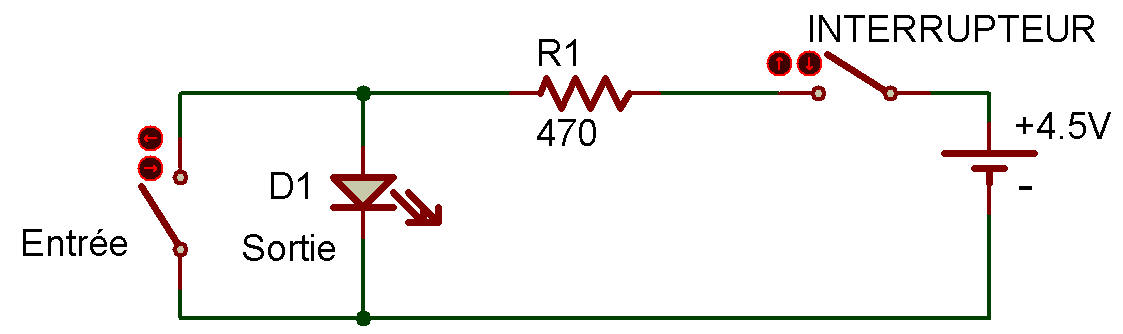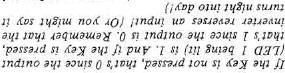An inverter circuitWe've mentioned digital electronics in earlier Projects, but what does the term mean? We've kept you in suspense long enough-it's time to find out. Digital circuits are also known as logic circuits. They are (if you'll excuse the pun!) "logical" because they can make decisions.

When we talk about digital circuits, we sometimes use the numbers 0 and 1. Number zero means the circuit is off while 1 means the circuit is on. All digital circuits are based on the simple fact that a circuit can be on or off.

Let's start out by exploring an inverter circuit. An inverter is a circuit that takes an input (an input is something that goes "into" a digital circuit) and "reverses" it.

When you finish wiring this Project, turn the power ON. LED 1 will light. This can be called 1. When you press the Key, LED 1 goes out. This is 0. But notice how the inverter "reverses" the input.

When there is no input (you don't press the Key), there is an output (LED 1 is lit), But if there is an input (you press the Key) there is no output (LED 1 goes out).

By the way, an output is something that "comes out of" a digital circuit.

Since an inverter reverses an input, what do you think the appropriate number would be if the Key is not pressed? If the Key is pressed? (No fair peeking at the answer?)Recherche personnalisée